#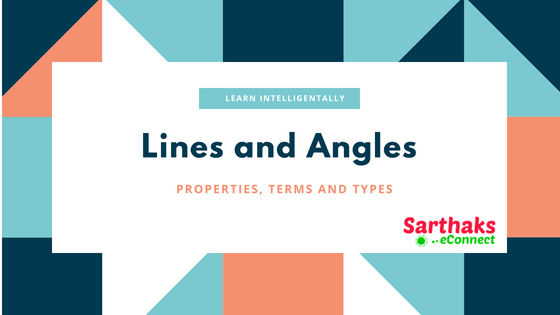Also read, Straight Line Circle Theorems Triangles Formula Conic Section Formula Coordinate Geometry

# Lines and Angles:

Do you ever think that the house you live in has a straight walls inclined ladder and much more thing is so much confined to its shape?

The reason is simple that is by the use of lines and angles everything wears a perfect shape. You now must aware that how lines and angles are important to us.

## Terminology related to lines and angles:

• Line: A collection of points which follows a straight path and can be drawn endlessly in both sides.
• Line Segment: A finite part of line is said to be line segment. It has two end-points.
• Ray: A line when cut at a point and its one end follows the infinite path is called ray. It has one end-point.
• Point of intersection: When two lines, we say they intersect; the meeting point is called the point of intersection.
• Angle: When two line or line segment intersects then at the intersecting point angles are formed.

## Terms related to angles:

• Complementary Angles: When the sum of the measures of two angles is 90°, the angles are called complementary angles.
Whenever two angles are complementary, each angle is said to be the complement of the other angle.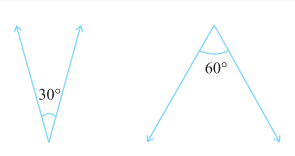These two angles are complementary.
• Supplementary Angles: When the sum of the measures of two angles is 180°, the angles are called complementary angles.
When two angles are supplementary, each angle is said to be the supplement of the other.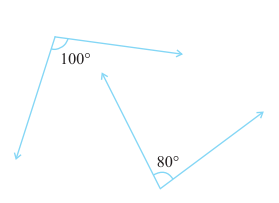These two angles are supplementary.
• Adjacent Angles: The condition of angle to be adjacent angles are they must have a common vertex, a common arm, and the non-common arms are on either side of the common arm.The angles 1 and two are adjacent angles.
• Linear Pair: A linear pair is a pair of adjacent angles whose non-common sides are opposite rays.
Also, say if sum of adjacent angle is 180⁰ then the angles are in linear pair.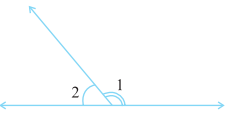Sum of angle 1 and 2 is 180⁰.
• Vertically Opposite Angles: When two lines intersect then the angles lying opposite the angle we took is called vertically opposite angles.
When two lines intersect, the vertically opposite angles so formed are equal.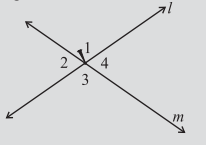Angle 1 & 3 and 2 & 4 are vertically opposite angles.

## Terms related to lines:

Intersecting Lines: When two lines are drawn on paper either intersect or when extended intersects is called intersecting lines.

Parallel lines: When two lines drawn on a paper doesn’t meet if extended to infinity is said to be parallel lines.

Transversal line: A line that intersects two or more distinct points is called transversal.
Example: p is a transversal to the lines l and m.## Angles Made by Transversal:Interior Angles ∠3, ∠4, ∠5, ∠6 Exterior Angles ∠1, ∠2, ∠7, ∠8 Pairs of Corresponding Angles ∠1 and ∠5, ∠2 and ∠6, ∠3 and ∠7, ∠3 and ∠7, ∠4and ∠8, Pairs of Alternate Interior Angles ∠3 and ∠6, ∠4 and ∠5, Pairs of Alternate Exterior Angles ∠1 and ∠8, ∠2 and ∠7, Pairs of Interior Angles on the same side of Transversal. ∠3 and ∠5, ∠4 and ∠6,

## Transversal of Parallel Lines:

• If two parallel lines are cut by a transversal then each pair of alternate interior angles are equal.
• If two parallel lines are cut by a transversal, then each pair of interior angles on the same side of the transversal are supplementary (i.e. sum of angle=180⁰).
• When a transversal cuts two lines, such that pairs of corresponding angles are equal, then the lines have to be parallel.